HOME

OVERVIEW

APPLICATIONS

•Geometry
•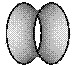Soap Bubbles
•Medical Imaging
•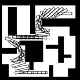Robotics
•Fluids
•Semiconductors
•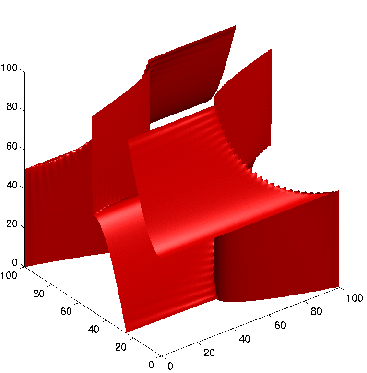Wave Propagation
•Image Denoising
•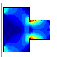Optimal Design
•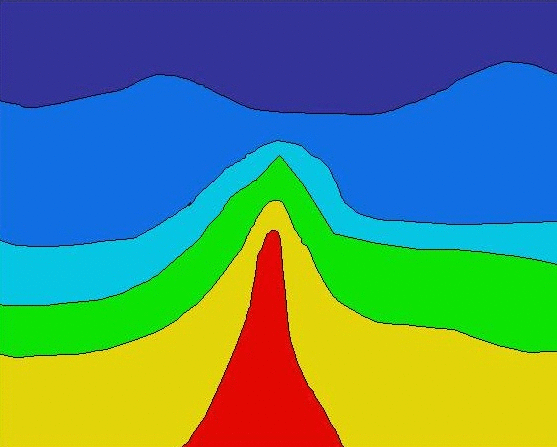Seismic Analysis
•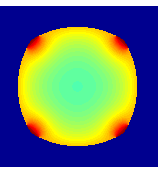Tumor Modeling
•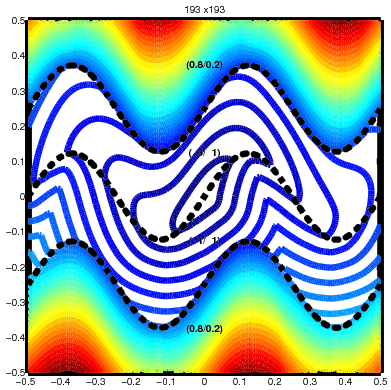Optimal Control
•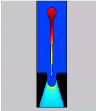InkJet Plotters
•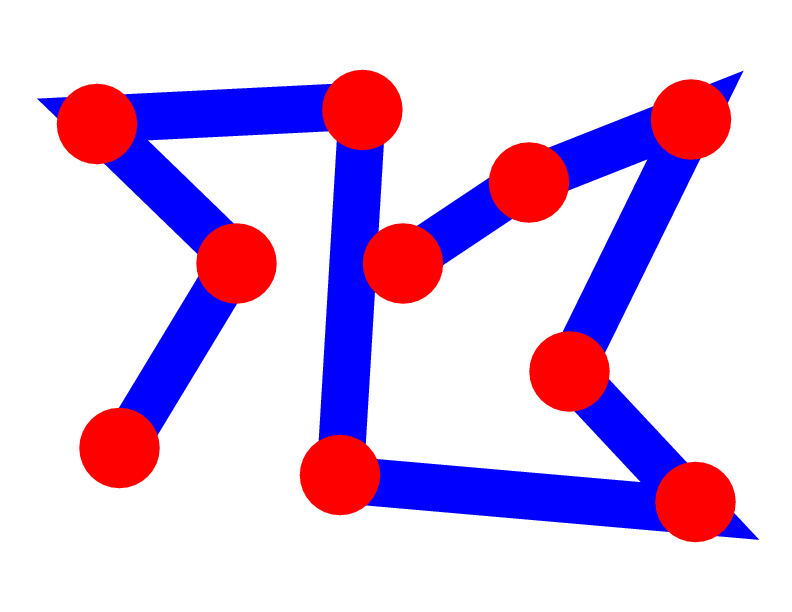Traveling Salesmen
•ViscoElastic Flow
•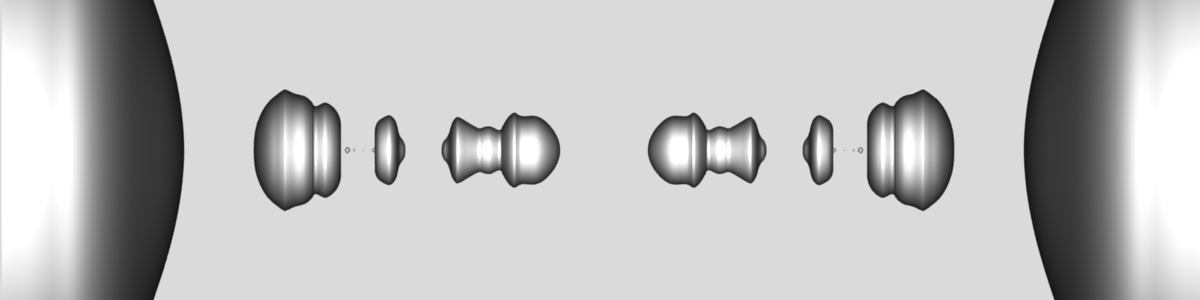Pinching Droplets
•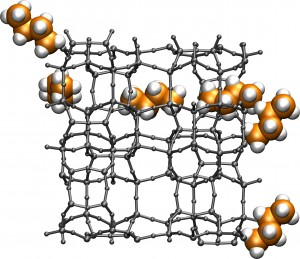Chemical Pathways

INTERACTIVE APPLETS

HISTORY OF THE METHODS/FLOW CHART

PUBLICATIONS

EDUCATIONAL MATERIAL

ACKNOWLEDGEMENTS

1996-2010
J.A. Sethian
• # Multiple Arrivals in Wave Propagation

### Motivation

Level set methods and Fast Marching Methods are designed to track a propagating interface, and find the first arrival of the interface as it passes a point. One way to think about this is to imagine that the front's boundary is a propagating flame that separates two regions: a burnt part behind the front and an unburnt part in front of the flame. Once a piece of ground is burnt, is burnt, it stays burnt: this corresponds to an entropy condition which insures that the motion is irreversible, and that the first arrival time when the disturbance hits is what is measured.

However, there are many situations, like propagating sound waves, in which later arrivals are also important. In the drawings below, a wave crosses over itself as it moves, creating both initial and later arrivals: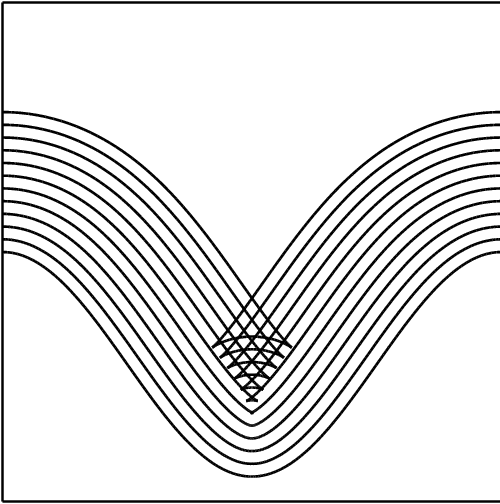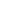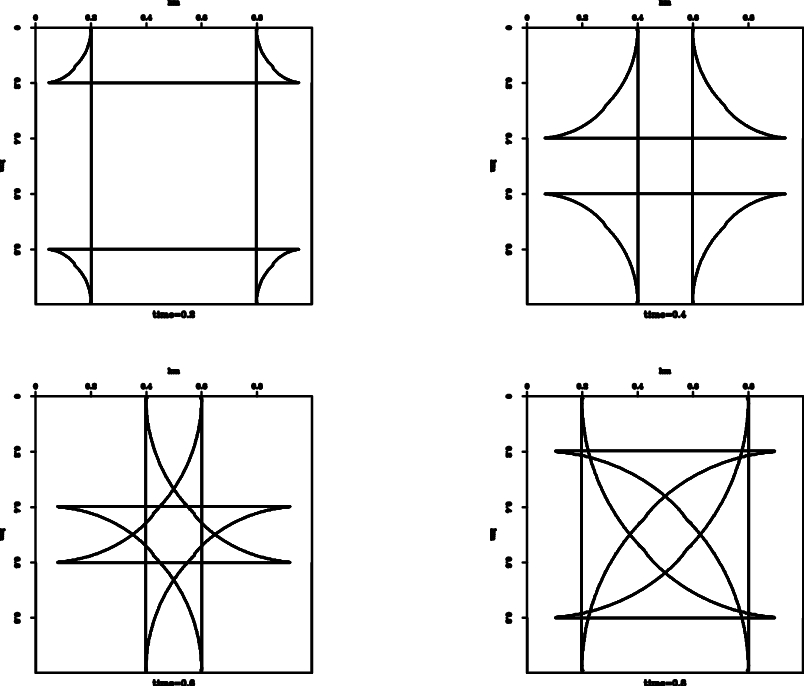Multiple arrivals from initial smooth curveMultiple arrivals from outer boundary

A good example of the important of these later arrivals is in geophysical imaging, in which one tries to predict what lies beneath the earth's surface by sending waves into the ground and recording their reflection. In this case, the first returning wave might not contain all the information, and later arrivals might contain more energy which can be used to more accurately predict what lies beneath.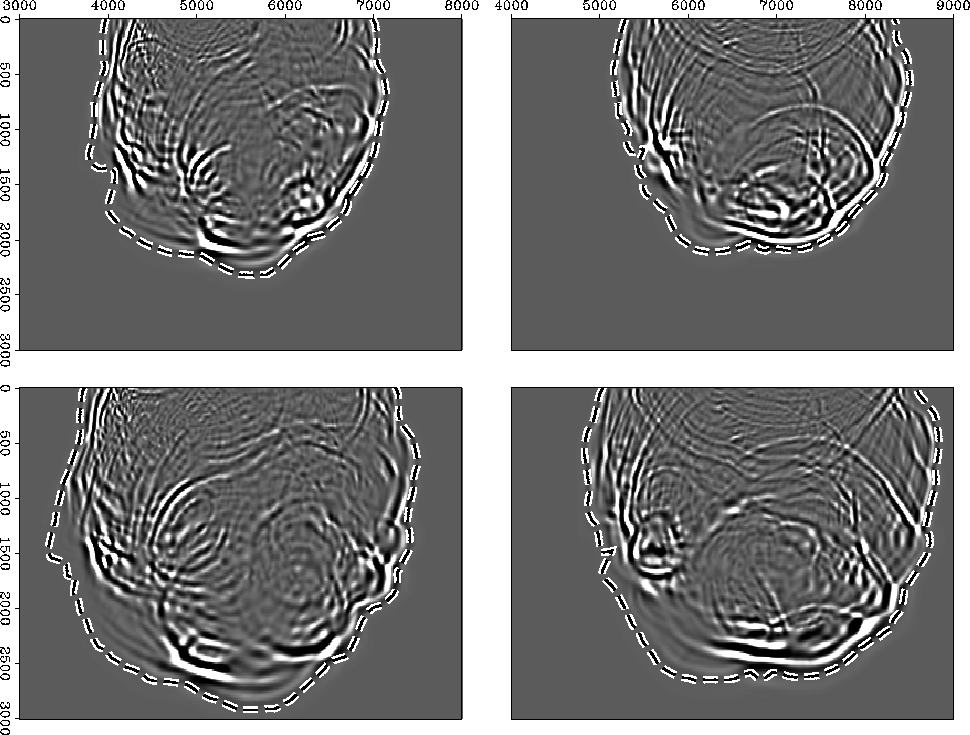Energy contained in many arrivals through a point

### Standard Approach

Suppose you want to compute the arrival of all waves starting at a source point, as in the figure on the left: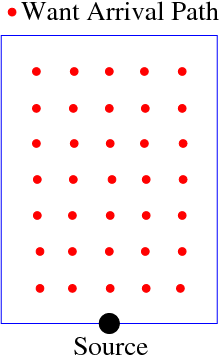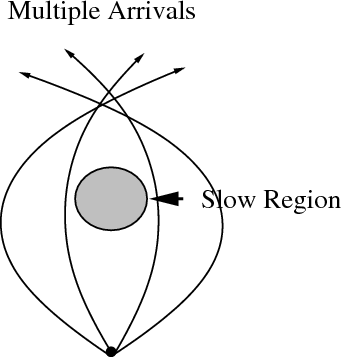Want arrivals at all the red pointsShoot rays from sourceRays diverge around slow region
You'll get the multiple arrivals for sure, but the rays won't be evenly balanced: they will cluster together as they pass around a fast region, and spread apart as they diverge around a slow region. As you might imagine, this becomes a tougher thing to do in three dimensions.

### A New, Evolving Interface Approach

Instead, we can transform this into a boundary value problem, and use something like the fast marching method, only this time, we will add an extra dimension to the problem. Let's start by thinking about a two dimensional problem. At each red spot in the domain, we can imagine three variables: the two x and y coordinates, and an additional coordinate theta corresponding to the takeoff angle from that point. Then we can imagine an arrow, leaving that point, passing and bending through the medium (the same way that light bends as passes through different media) and landing somewhere on the boundary: the place and direction it lands are called escape coordinates.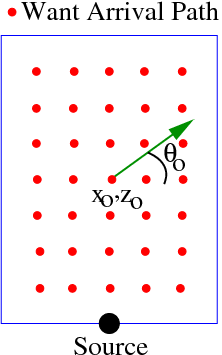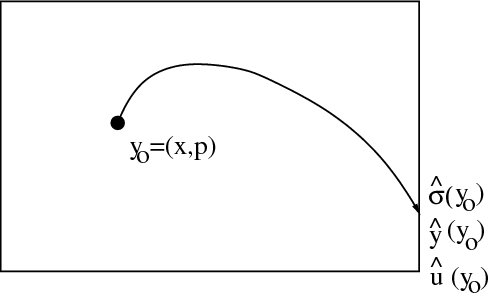We can write down a set of equations for the escape coordinates: what's great is that they don't contain time anymore, and that means we need only solve a boundary value problem, similar to the Eikonal

equation (though with an extra dimension). We can do this by solving using a variant of fast marching methods and ordered upwind methods. Start a surface at the boundary (in three dimensional phase space): for every point on this boundary, we know the escape position and angle, since it is the same as the starting point (it's already on the exit!). Then, we can systematically march inwards, reaching back to the known escape values, and eventually cover the entire phase space cube: this is what is shown below: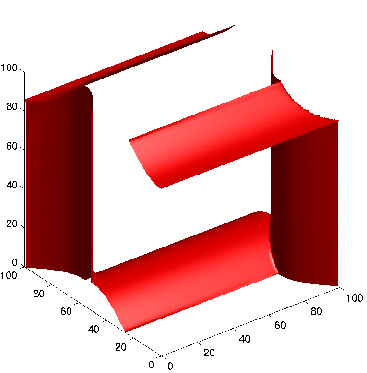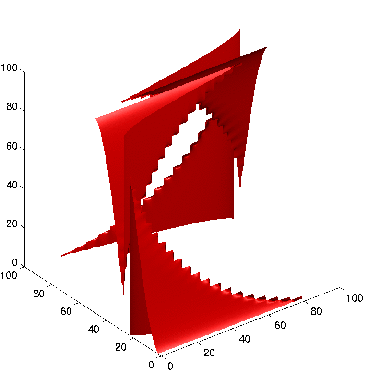Evolving Surface of Known Escape Values: T=.3 Evolving Surface of Known Escape Values: T=1.0 Evolving Surface of Known Escape Values: T=2.0

### Results

Below is one result from this technique: Waves propagate from the top point through a region that contains a slowness disk in the center: you can easily see the waves propagate around the slow part, and double back on themselves, creating multiple arrivals.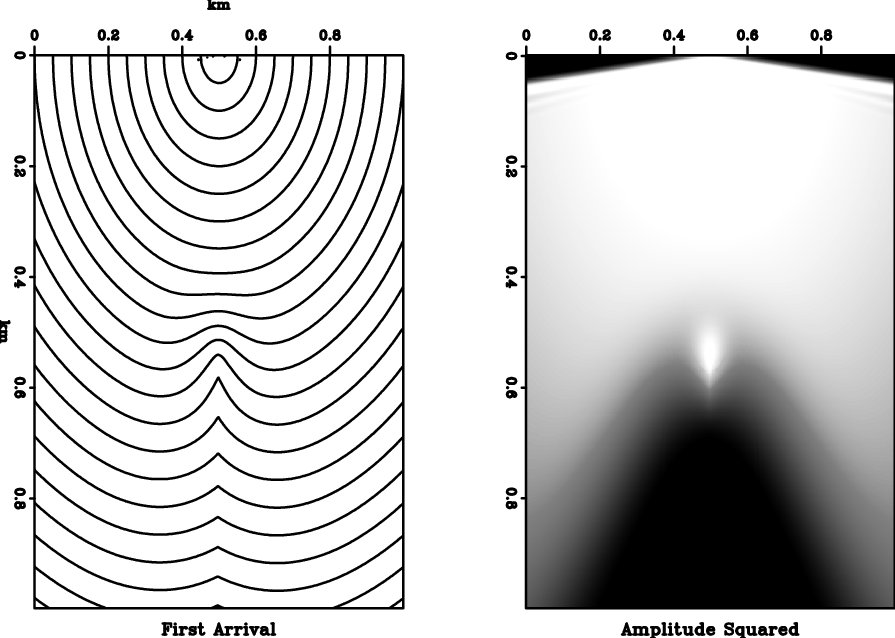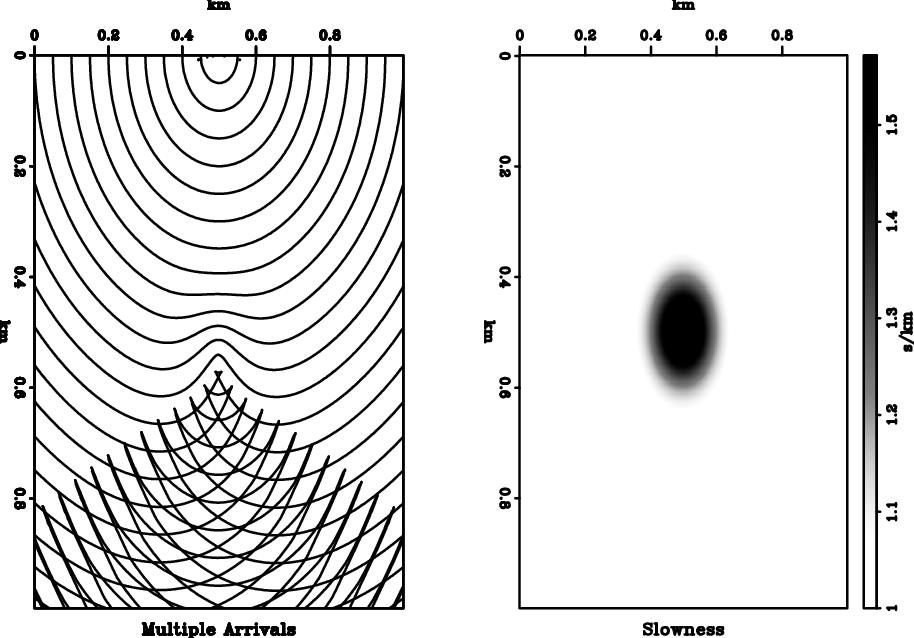### Details

We developed a fast, general computational technique for computing the phase-space solution of static Hamilton-Jacobi equations. Starting with the Liouville formulation of the characteristic equations, we derived ``Escape Equations'' which are static, time-independent Eulerian PDEs. They represent all arrivals to the given boundary from all possible starting configurations. The solution is numerically constructed through a `one-pass' formulation, building on ideas from semi-Lagrangian methods, Dijkstra-like methods for the Eikonal equation, and Ordered Upwind Methods. To compute all possible trajectories corresponding to all possible boundary conditions, the technique is of computational order O(N \log N), where N is the total number of points in the computational phase-space domain; any particular set of boundary conditions is then extracted through rapid post-processing. The technique can be applied to the problem of computing first, multiple, and most energetic arrivals to the Eikonal equation.